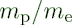## Sunday, August 14, 2016

### Another Equation for Proton to Electron Mass Ratio???

$$\mu={m_p\over m_e}={2\phi m_{\ell}\over {\phi_em_{\ell}/2\alpha}}=4\alpha{\phi\over\phi_e}=4\alpha{a_0\over r_p}=1836.15267...$$
Compare to:
$$\mu={\alpha^2\over{\pi r_pR_{\infty}}}=1836.15267...$$
$\mu=$ proton to electron  mass ratio
$m_p=$ mass of proton
$m_e=$ mass of electron
$\phi={\eta\over R}$ Holographic ratio for proton
$\phi_e={\eta_e\over R_e}$ Holographic ratio for electron
$m_{\ell}=$ Planck mass
$a_0=$ Bohr radius
$r_p=$ proton radius (muonic hydrogen proton radius)
$R_{\infty}=$ Rydberg constant

((4 * fine-structure constant * hbar) / (m_e * c * fine-structure constant)) / (((4 * hbar) / c) / m_p) =
1 836.15267

CODATA value for proton-electron mass ratio:
http://physics.nist.gov/cgi-bin/cuu/Value?mpsme
 proton-electron mass ratioValue 1836.152 673 89Standard uncertainty 0.000 000 17Relative standard uncertainty 9.5 x 10-11Concise form 1836.152 673 89(17)

There remain a few issues to be ironed out as Haramein's paper, The Electron and the Holographic Mass Solution, reports the proton to electron mass ratio as:
$$\mu={m_p\over m_e}={2\phi m_{\ell}\over {\phi_em_{\ell}/2\alpha}}=4\alpha{\phi\over\phi_e}=1836.942579077855...$$

So, the work continues to understand this difference...

This result, the 1836.94259077855... above, is calculated using the CODATA value for $\alpha$, the 2013 muonic hydrogen charge radius in the $phi$ calculation, and $phi_e=6.108458512E-25$ .  See Google Drive Excel File for calculations.

More in upcoming post: Another Equation for Proton to Electron Mass Ratio!!! #2

The Surfer, OM-IV